# Array Representation of Sparse Matrix

## Array Representation of Sparse Matrix:

In this article, I am going to discuss the Array Representation of the Sparse Matrix in C and C++ Language with Examples. Please read our previous article, where we discussed the Addition of Sparse Matrices. In this article, we will discuss the following pointers.

1. How to Represent a Sparse Matrix?
2. How to Create a Sparse Matrix?
3. How to Add Two Sparse Matrices?

Instead of a 2D array, we will represent the sparse matrix by using a coordinate list or the 3-column method. Let us start with representation.

##### Representation of Sparse Matrix:

Now, let’s see the representation of the sparse matrix. The non-zero elements in the sparse matrix can be stored using triplets that are rows, columns, and values. There are two ways to represent the sparse matrix that are listed as follows –

1. Array Representation
##### Array Representation of the Sparse Matrix

Representing a sparse matrix by a 2D array leads to the wastage of lots of memory. This is because zeroes in the matrix are of no use, so storing zeroes with non-zero elements is a waste of memory. To avoid such wastage, we can store only non-zero elements. If we store only non-zero elements, it reduces the traversal time and the storage space.

##### Representation of Sparse Matrix: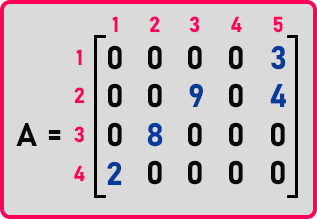This is the matrix that we want to implement in code. In the previous article, we have seen a table that contains the non-zero element as well as their column and row number. Below is the table for matrix ‘A’: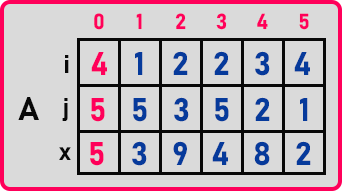0th column contains the dimension of matrix-like ‘4’ rows, ‘5’ columns, and ‘5’ non-zero elements. And all the other columns contain the non-zero elements like 1st row, 5th column and element are ‘3’. So, for each non-zero element we require 3 things that are:

1. The row number of that element
2. Column number of that element
3. The element

We are calling row number as ‘i’, column number as ‘j’, and element as ‘x’. So, we can combine these 3 values and make a structure. Let us define a structure called ‘element’ as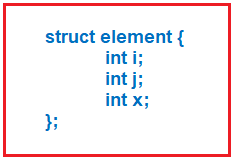This is the structure for an element of the matrix. It contains ‘i’ as row number, ‘j’ as column number and ‘x’ as the value of the element. So, it defines a single non-zero element of a sparse matrix. To implement the above table, we need an array of elements. In the above matrix, there are 5 non-zero elements so we require an array of size ‘5’. Now let us define the structure for the sparse matrix.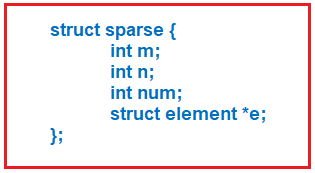Here ‘m’ is no. of rows, ‘n’ is no. of columns, and ‘num’ is no. of non-zero elements. We will use this structure to store the dimension and no. of non-zero elements of a sparse matrix. For the rest of the elements, we can define them as an array of elements. So ‘e’ is a type of ‘element *’. It will hold the values of non-zero elements.

Should we have declared it as an array of some size? No, if we declare an array of some size then it will be limited to some elements. Instead of taking static size, let us take it as a dynamic size array so for that we have made it as a pointer. Now let us see how to use this structure and create a sparse matrix.In our main function of the program, we have declared an object of sparse. This object has,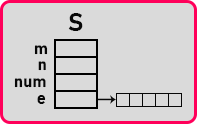Now we will create a ‘create’ function to create the sparse matrix.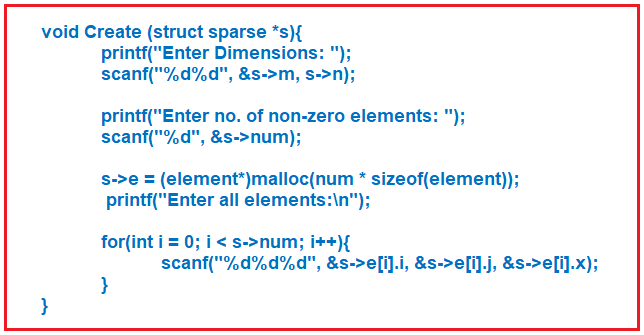So, this is the code to create a sparse matrix by taking input from the user. Let us look at the complete program.

##### Program to Add 2 Sparse Matrices using C Language:
```#include <stdio.h>
#include<stdlib.h>
struct Element
{
int i;
int j;
int x;
};
struct Sparse
{
int m;
int n;
int num;
struct Element *ele;
};

void create (struct Sparse *s)
{
int i;
printf ("Eneter Dimensions: ");
scanf ("%d%d", &s->m, &s->n);
printf ("Number of non-zero: ");
scanf ("%d", &s->num);

s->ele = (struct Element *) malloc (s->num * sizeof (struct Element));
printf ("Eneter non-zero Elements:\n");

for (i = 0; i < s->num; i++)
scanf ("%d%d%d", &s->ele[i].i, &s->ele[i].j, &s->ele[i].x);
}

void display (struct Sparse s)
{
int i, j, k = 0;
for (i = 0; i < s.m; i++)
{
for (j = 0; j < s.n; j++)
{
if (i == s.ele[k].i && j == s.ele[k].j)
printf ("%d ", s.ele[k++].x);
else
printf ("0 ");
}
printf ("\n");
}
}

struct Sparse *add (struct Sparse *s1, struct Sparse *s2)
{
struct Sparse *sum;
int i, j, k;
i = j = k = 0;

if (s1->n != s2->n && s1->m != s2->m)
return NULL;

sum = (struct Sparse *) malloc (sizeof (struct Sparse));
sum->ele = (struct Element *) malloc ((s1->num + s2->num) * sizeof (struct Element));

while (i < s1->num && j < s2->num)
{
if (s1->ele[i].i < s2->ele[j].i)
sum->ele[k++] = s1->ele[i++];
else if (s1->ele[i].i > s2->ele[j].i)
sum->ele[k++] = s2->ele[j++];
else
{
if (s1->ele[i].j < s2->ele[j].j)
sum->ele[k++] = s1->ele[i++];
else if (s1->ele[i].j > s2->ele[j].j)
sum->ele[k++] = s2->ele[j++];
else
{
sum->ele[k] = s1->ele[i];
sum->ele[k++].x = s1->ele[i++].x + s2->ele[j++].x;
}
}
}
for (; i < s1->num; i++)
sum->ele[k++] = s1->ele[i];
for (; j < s2->num; j++)
sum->ele[k++] = s2->ele[j];
sum->m = s1->m;
sum->n = s1->n;
sum->num = k;
return sum;
}

int main()
{
struct Sparse s1, s2, *s3;
printf ("Enter First Matrix\n");
create (&s1);
printf ("Enter Second Matrix\n");
create (&s2);

printf ("First Matrix\n");
display (s1);
printf ("Second Matrix\n");
display (s2);
printf ("Sum Matrix\n");
display (*s3);

return 0;
}
```
###### Output: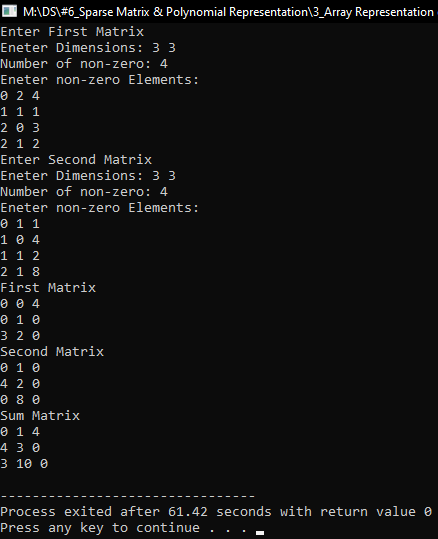##### Sparse Matrix implementation in C++:
```#include <iostream>
using namespace std;
class Element
{
public:
int i;
int j;
int x;
};

class Sparse
{
private:
int m;
int n;
int num;
Element *ele;
public:
Sparse (int m, int n, int num)
{
this->m = m;
this->n = n;
this->num = num;
ele = new Element[this->num];
}
~Sparse ()
{
delete[]ele;
}

Sparse operator + (Sparse & s);

friend istream & operator >> (istream & is, Sparse & s);
friend ostream & operator << (ostream & os, Sparse & s);
};

Sparse Sparse::operator + (Sparse & s)
{
int i, j, k;
if (m != s.m || n != s.n)
return Sparse (0, 0, 0);
Sparse *sum = new Sparse (m, n, num + s.num);

i = j = k = 0;

while (i < num && j < s.num)
{
if (ele[i].i < s.ele[j].i)
sum->ele[k++] = ele[i++];
else if (ele[i].i > s.ele[j].i)
sum->ele[k++] = s.ele[j++];
else
{
if (ele[i].j < s.ele[j].j)
sum->ele[k++] = ele[i++];
else if (ele[i].j > s.ele[j].j)
sum->ele[k++] = s.ele[j++];
else
{
sum->ele[k] = ele[i];
sum->ele[k++].x = ele[i++].x + s.ele[j++].x;
}
}
}

for (; i < num; i++)
sum->ele[k++] = ele[i];
for (; j < s.num; j++)
sum->ele[k++] = s.ele[j];
sum->num = k;

return *sum;
}

istream & operator >> (istream & is, Sparse & s)
{
cout << "Enter non-zero elements:\n";
for (int i = 0; i < s.num; i++)
cin >> s.ele[i].i >> s.ele[i].j >> s.ele[i].x;
return is;
}

ostream & operator << (ostream & os, Sparse & s)
{
int k = 0;
for (int i = 0; i < s.m; i++)
{
for (int j = 0; j < s.n; j++)
{
if (s.ele[k].i == i && s.ele[k].j == j)
cout << s.ele[k++].x << " ";
else
cout << "0 ";
}
cout << endl;
}
return os;
}

int main ()
{
Sparse s1 (3, 3, 4);
Sparse s2 (3, 3, 4);

cout << "Enter First Matrix:" << endl;
cin >> s1;
cout << "Enter Second Matrix:" << endl;
cin >> s2;

Sparse sum = s1 + s2;

cout << "First Matrix" << endl << s1;
cout << "Second MAtrix" << endl << s2;
cout << "Sum Matrix" << endl << sum;

return 0;
}
```
###### Output: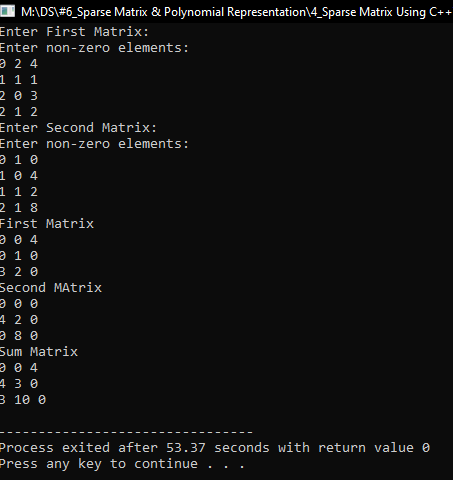In the next article, I am going to discuss Polynomial Representation, Evaluation, and Addition with Examples using C Language. Here, in this article, I try to explain the Array Representation of Sparse Matrix in C and C++ Language with Examples and I hope you enjoy this Array Representation of Sparse Matrix in C and C++ Language article.